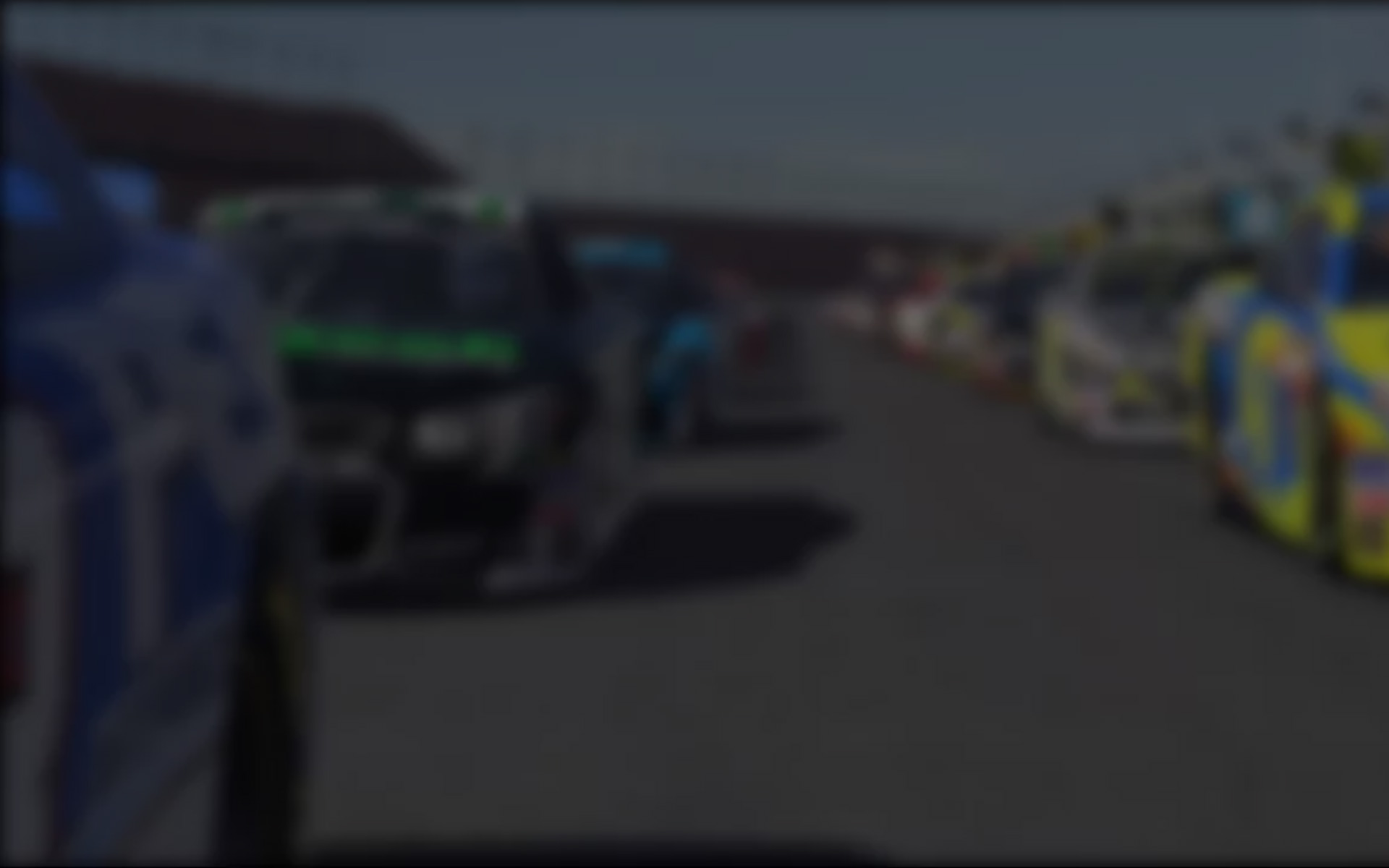Lately I have been running the engine so hard in the Skip Barber F2000 that it wails in such a way that I think I need to call Father Karras. In the past I was kind and gentle to the powertrain, but now I’ve learned that it needs to be pushed closer to the limit to be faster.

As hinted in a previous tech tip, I ran some tests at Indianapolis to determine approximate power and torque curves. This is easiest to show using the Skip Barber since it is a straightforward test in this car, as the car is very easily brought up to full speed and handles very neutral with few complicating setup factors.

If you have a telemetry logger like vbox, this testing involves starting the car at the beginning of a straight in a single gear and winding it out without shifting until it hits the rev limiter or the car reaches the end of the straight or its terminal velocity. Repeat this for each gear and then do another test where you bring-up the car to maximum speed near the beginning of a straight, push-in the clutch and then let it coast down to a stop.

With the data in hand, you can begin by calculating the change in speed divided by the change in time to show the instantaneous acceleration rate. After you plot this versus speed for the coasting data, you now can then apply a trendline (2nd power) and use the resulting equation to estimate the resistance acceleration for each speed data point in the acceleration runs. When you add the actual acceleration rate to the resistance acceleration (thus subtracting the losses due to aerodynamic drag and rolling resistances), you will find an approximation of the true acceleration to the wheels. This can be converted to power by the following equation:

Power to Wheels = mass x total acceleration x velocity

If you assume that drivetrain losses are roughly 17%, you can get a fairly good estimate of what the power curve would look like on a dyno.

And torque in metric units can also be derived using:

Torque = Power x 60 / (2 x pi x RPM)

If you take care of units (for simplicity I do everything in metric and then convert to HP and ft-lb at the end), you can plot the Power to wheels vs RPM. The example shown is for the Skip in 4th gear.We can also plot Power vs Speed to show how each gear will interact with the next. Also shown is the resistance from aerodynamic drag and mechanical/rolling resistance. Thus we now have a clear indication of what the optimal shift points are and the maximum power during acceleration through the gears is depicted by the dotted green line. Of course, shifts are not instantaneous, but this is made moot by flat-shifting (full throttle during the shift which most everyone does in the sim, but which would be frowned upon in some of these cars in real life). Flat shifting winds-up the engine speed while in neutral and when re-engaged in the next gear causes a sudden surge in acceleration which covers up the loss while in neutral. The end result of the flat-shift in the Skip Barber car is almost no loss of speed from the earlier progression in the previous gear.But studying where the power curves intersect shows where the loss of acceleration is minimized and, when determining the corresponding RPM, it is surprising (at least to me) that the best results will occur when the car is shifted close to the rev limit of 6400 RPM.

But to shift so close to the rev limit in first gear is extremely tricky since the RPMs are changing so rapidly. So I prescribe the following upshift points for the Skip Barber car based on the analysis of the data:

1st – 2nd : 6200 RPM (reduced from optimal to avoid triggering the rev limiter)
2nd – 3rd : 6200 RPM
3rd – 4th : 6100 RPM
4th – 5th : 6300 RPM

Warning – 6300 RPM will sound like Crazy Frog. Do not be alarmed! On the next installment I’ll compare optimal shift RPMs with non-optimal to measure the difference in performance.

Now you may find me driving the Skip like a man possessed. Say a prayer for me.